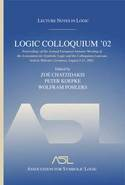# Lecture Notes in Logic, 27

## Logic Colloquium ’02

Zoé Chatzidakis, Peter Koepke, Wolfram Pohlers, editorsYear: 2006

ISBN: 1-56881-300-7
370 pages. Hardcover.

Logic Colloquium ’02 includes articles from some of the world’s preeminent logicians. The topics span all areas of mathematical logic, but with an emphasis on Computability Theory and Proof Theory. This book will be of interest to graduate students and researchers in the field of mathematical logic

• Davod Asperó
Generic absoluteness for \$\Sigma_1\$ formulas and the continuum problem
• Joan Bagaria
Axioms of generic absoluteness
• Arnold Beckmann
Generalised dynamic ordinals—universal measures for implicit computational complexity
• Lev D. Beklemishev
The Worm principle
• Johan van Benthem
“One is a lonely number”:logic and communication
• Vasco Brattka
Computable versions of the uniform boundedness theorem
• Elías F. Combarro
Symmetry of the universal computable function: A study of its automorphisms, homomorphisms and isomorphic embeddings
• Moti Gitik, Ralf Schindler, and Saharon Shelah
PCF theory and Woodin cardinals
• Steffen Lempp, Manuel Lerman, and Reed Solomon
Embedding finite lattices into the computably enumberable degrees—a status survey
• Olivier Lessmann
Dimension theory insided a homogeneous model
• André Nies
Reals which compute little
• Martin Otto
Bisimulation invariance and finite models
• Michael Rathjen
Choice principles in constructive and classical set theories
• Ivan N. Soskov and Vessela Baleva
Ash’s theorem for abstract structures
• Frank Stephan
Martin-Löf random and PA-complete sets
• Sebastiaan A. Terwijn
Learning and computing in the limit